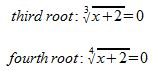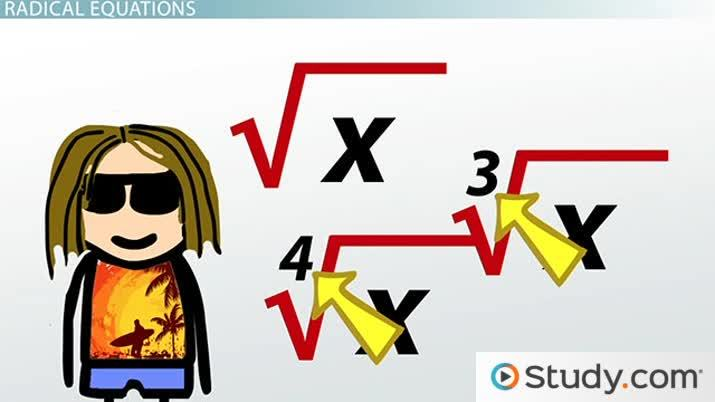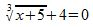Lesson Transcript
Instructor: Yuanxin (Amy) Yang Alcocer

Amy has a master's degree in secondary education and has been teaching math for over 9 years. Amy has worked with students at all levels from those with special needs to those that are gifted.

Radical equations can be solved after changing them into linear or quadratic equations. Learn how to solve radical equations by removing the radical and changing them into linear or quadratic equations. Updated: 10/02/2021

If you see an equation like the square root of (x + 2) = 0, you can say, 'That's so radical!' Do you see the symbol that the square root uses? In math, we call that the radical symbol. Besides the square root, we can also have third roots, fourth roots, and so on.

What do these mean? While the square root tells you to look for something that if you multiply it together twice you will get the number inside the square root symbol, a third root tells you to look for something that if you multiply it together three times, you will get the number inside the radical. Can you guess how the fourth root works? Yes, a fourth root is a number where if you multiply it together four times, you will get your number inside the radical.

So, how do these look? Well, you know what the square root symbol looks like. The third root and the fourth root also use the same symbol except that now you will see a little number to the upper left of the symbol like this.Do you see the little number right where the symbol makes a dip? That number tells you how many times to multiply your answer to get the number inside the radical. If you don't see a number, then you are looking at a square root that has an invisible little two there.An error occurred trying to load this video.

Try refreshing the page, or contact customer support.

Coming up next: Squaring Both Sides of an Equation Twice

### You're on a roll. Keep up the good work!

Replay
Your next lesson will play in 10 seconds
• 1:18 How to Remove Radicals
• 2:43 Change to Linear Equation
• 3:33 Change to Quadratic Equation
• 4:31 Lesson Summary
Save Save

Want to watch this again later?

Timeline
Autoplay
Autoplay
Speed Speed

How would you solve something like this? What do you think? We would want to remove the radical somehow so that we could get the x so we can solve for it. So how do we do that? Let me show you.

What we need to do first is to look for the little number if it is there. If there is no number, then the number is 2. It is this little number that holds the key to removing the radical. If you think of the radical symbol as a lock, then this little number tells you the password that will unlock it.

Okay, so how do we unlock it? We unlock it by multiplying the side of the equation with the radical by itself as many times as that little number tells us. In other words, we would take that side of the equation to the power that the little number tells us to.

For a square root, we square it; for a third root, we cube it; for a fourth root, we take it to the fourth power, and so on and so forth. We also remember that if we do one operation on one side of the equation, we have to do it to the other side as well.

Also, if you have an equation such as sqrt(x + 2) - 1 = 0, you would want to move the -1 over to the other side of the equation first so that your radical is by itself before you unlock the radical. Let's take a look at a couple of examples.

## Change to a Linear Equation

First, if inside our radical, we have an x, we will end up with a linear equation after removing the radical. Let's solve this equation.To unlock this lesson you must be a Study.com Member.

### Register to view this lesson

Are you a student or a teacher?